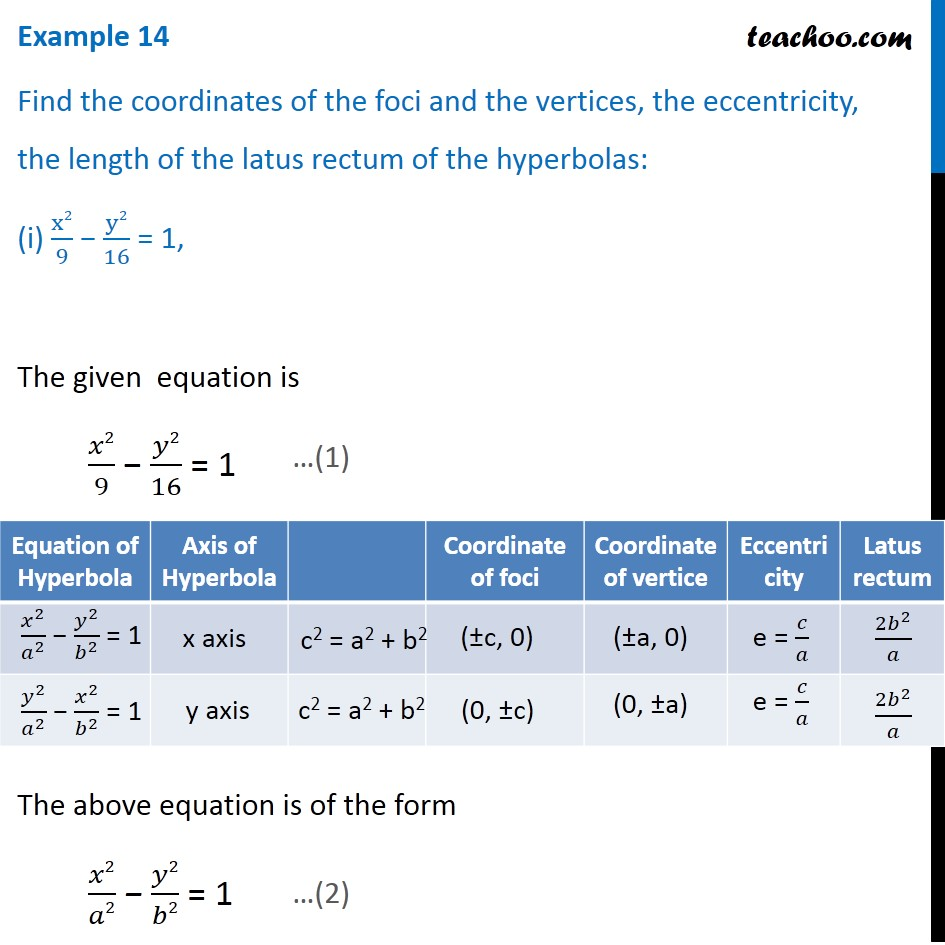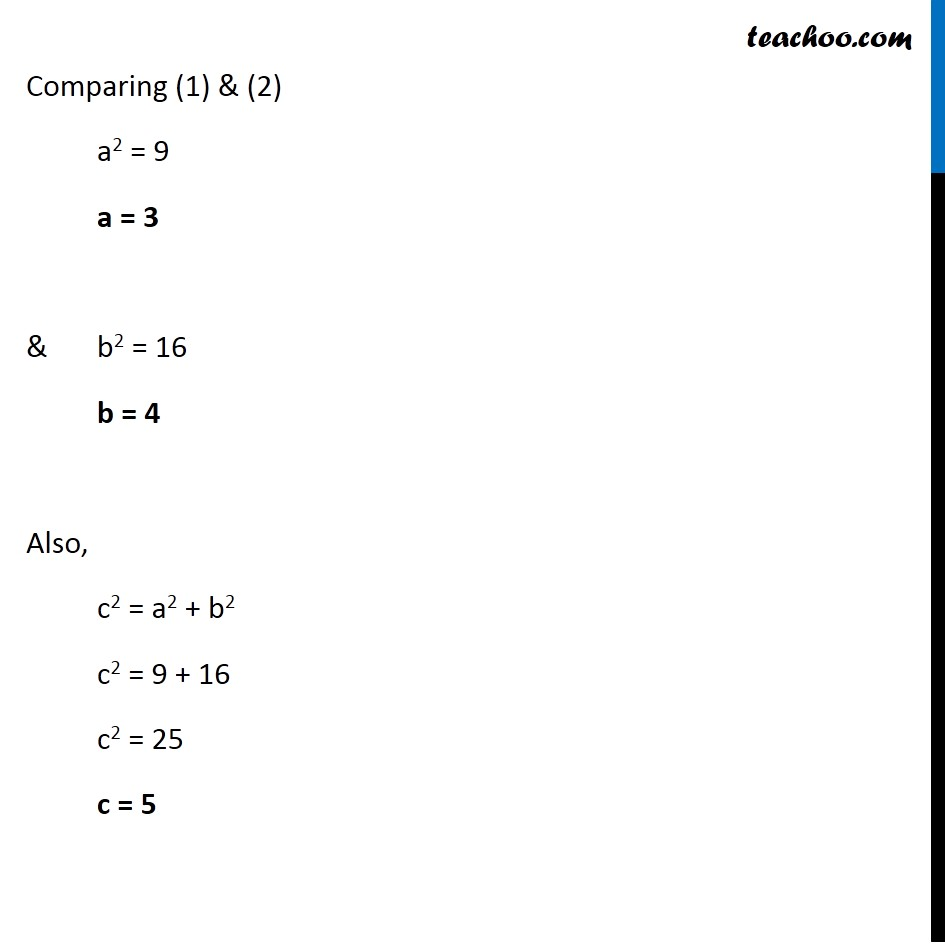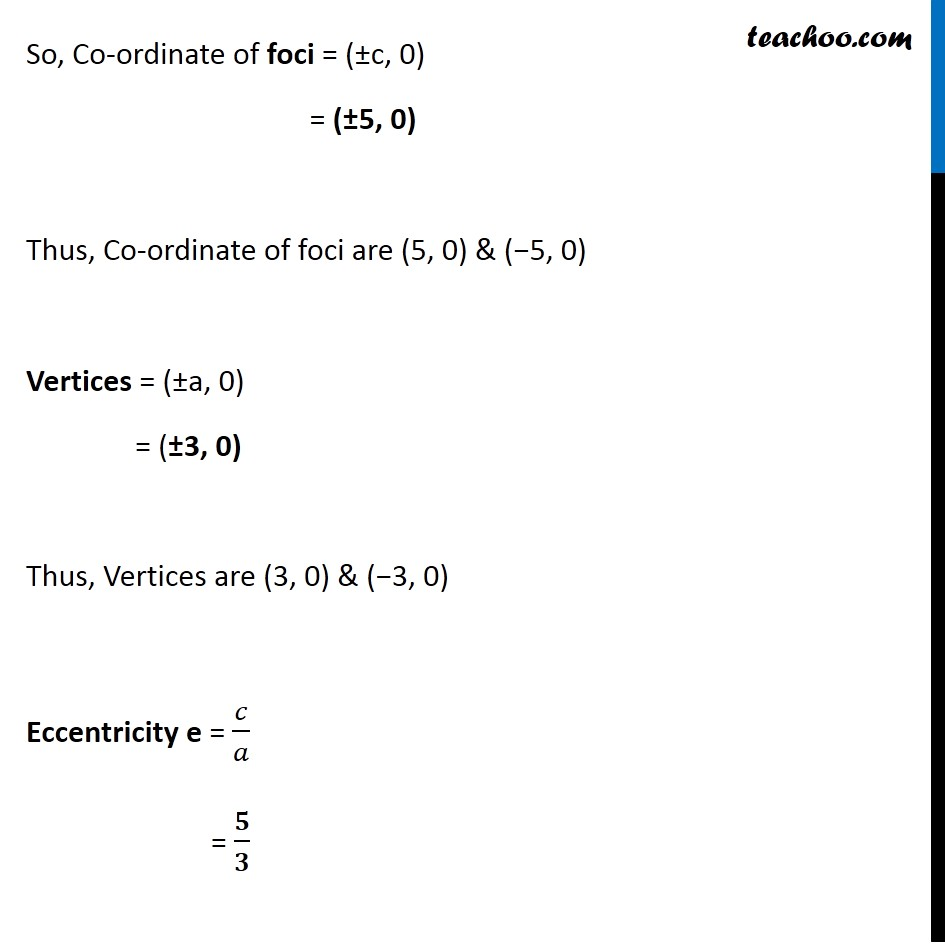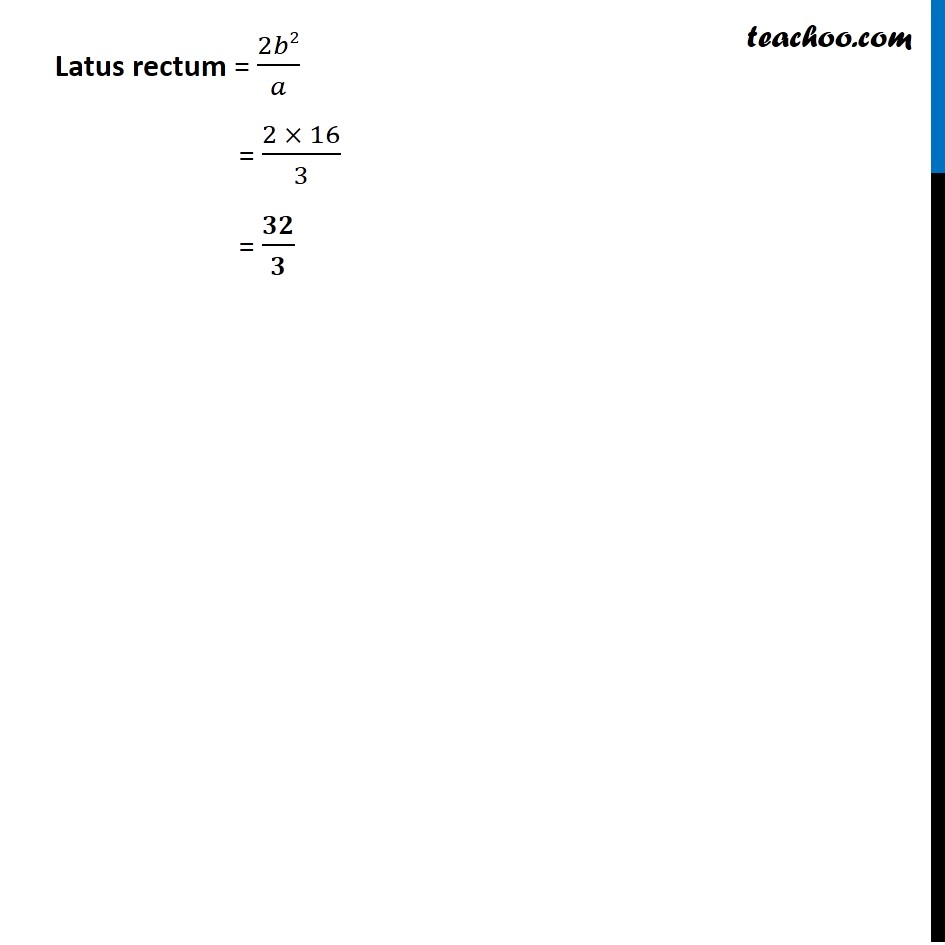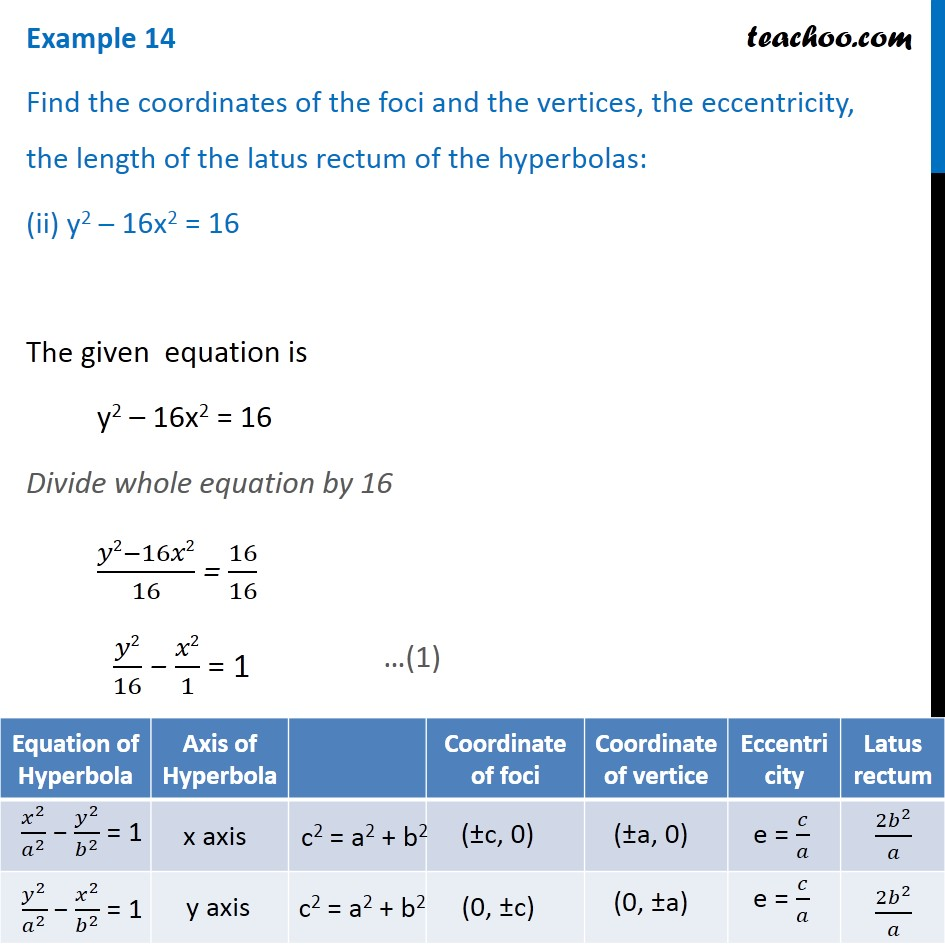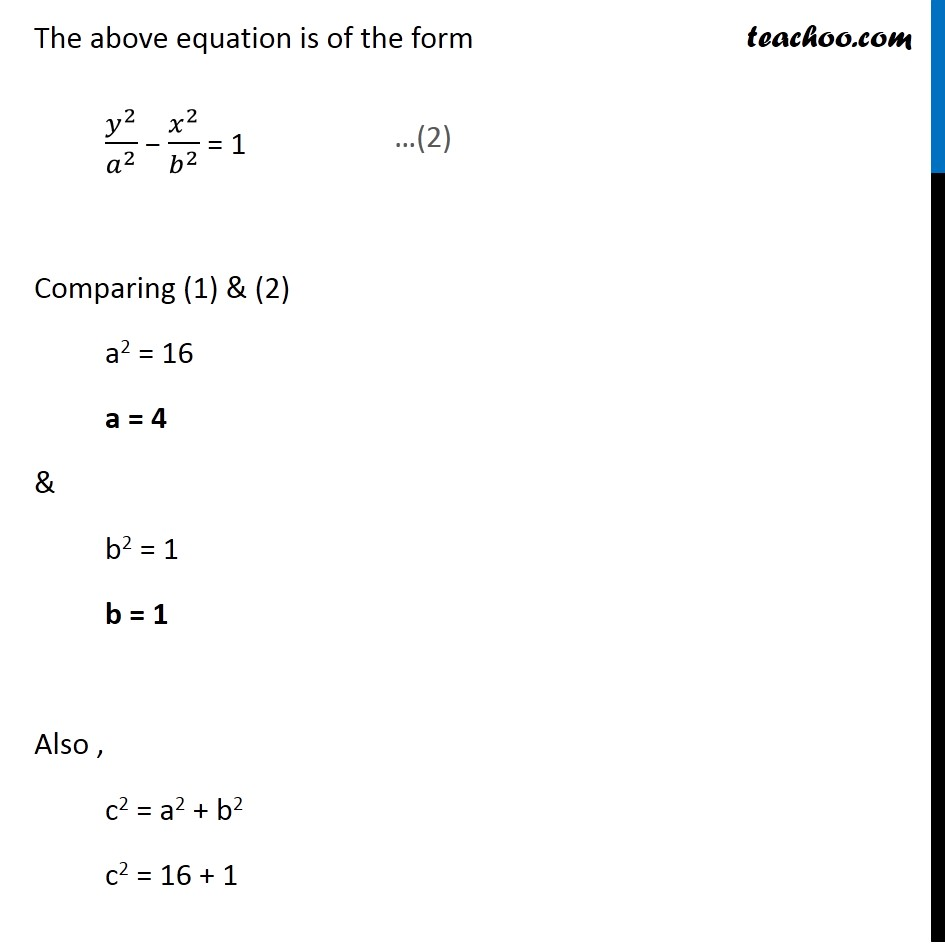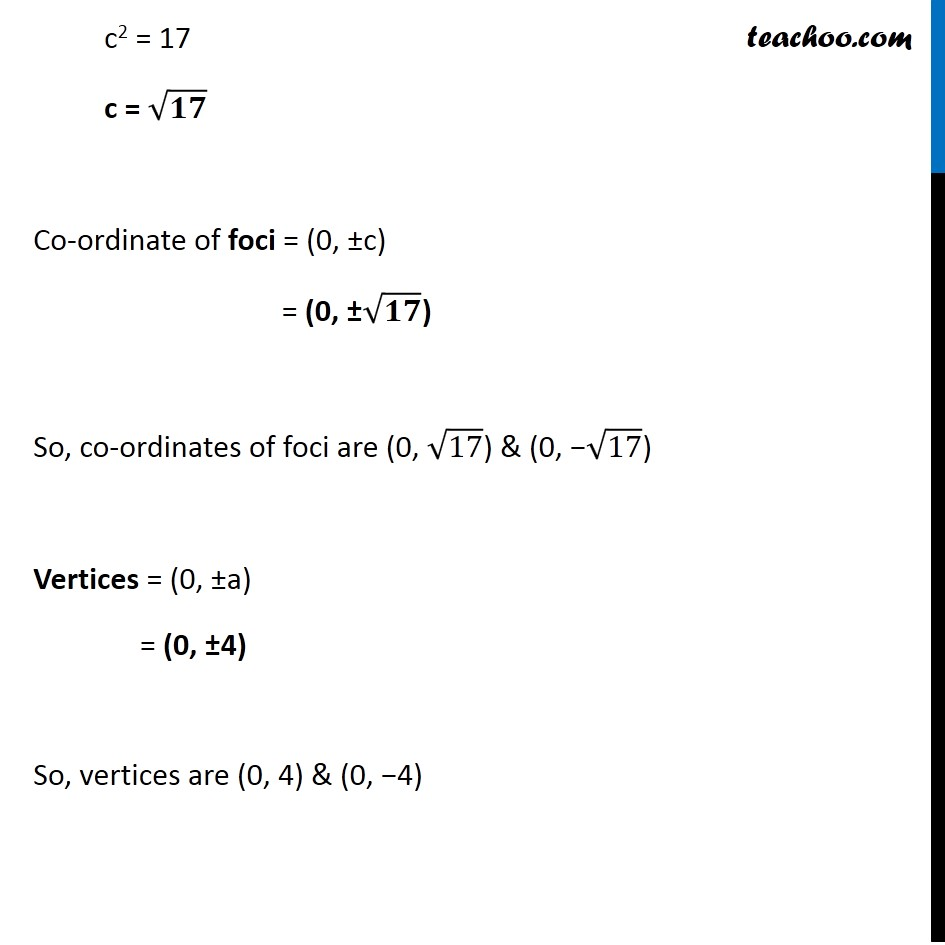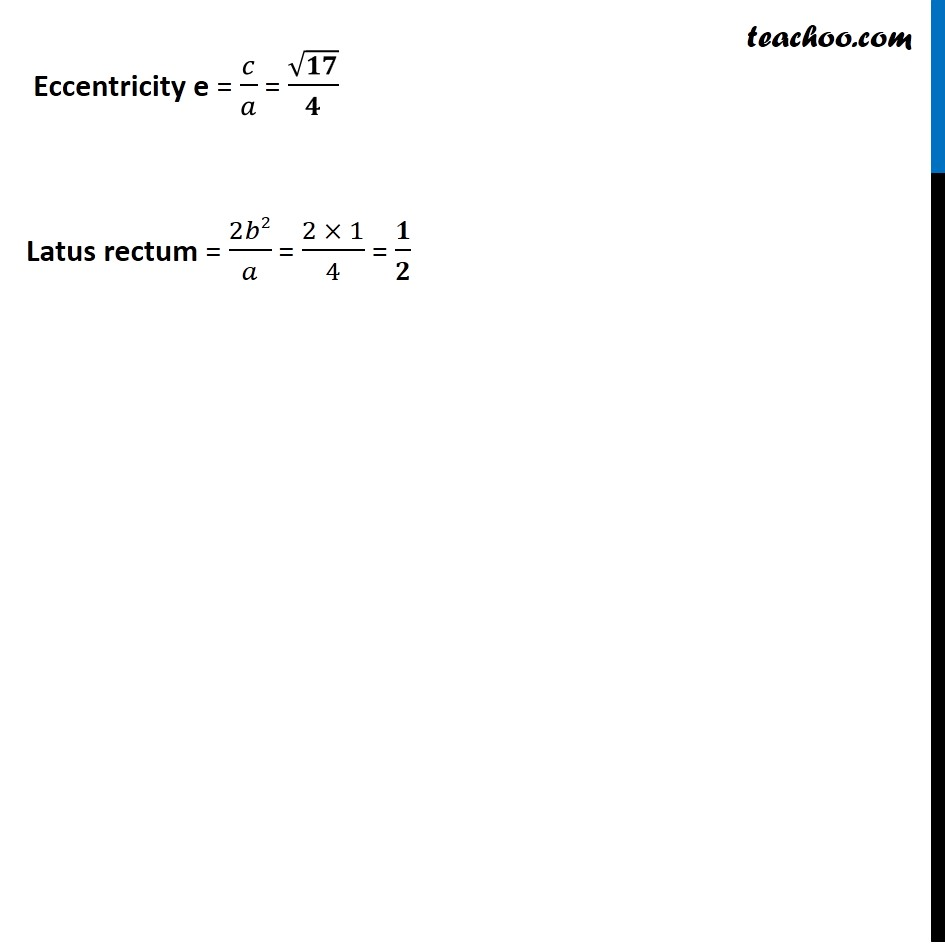Subscribe to our Youtube Channel - https://you.tube/teachoo

1. Chapter 11 Class 11 Conic Sections
2. Serial order wise
3. Examples

Transcript

Example 14 Find the coordinates of the foci and the vertices, the eccentricity, the length of the latus rectum of the hyperbolas: (i) x2/9 − y2/16 = 1, The given equation is 𝑥2/9 − 𝑦2/16 = 1 The above equation is of the form 𝑥2/𝑎2 − 𝑦2/𝑏2 = 1 Comparing (1) & (2) a2 = 9 a = 3 & b2 = 16 b = 4 Also, c2 = a2 + b2 c2 = 9 + 16 c2 = 25 c = 5 So, Co-ordinate of foci = (±c, 0) = (±5, 0) Thus, Co-ordinate of foci are (5, 0) & (−5, 0) Vertices = (±a, 0) = (±3, 0) Thus, Vertices are (3, 0) & (−3, 0) Eccentricity e = 𝑐/𝑎 = 𝟓/𝟑 Latus rectum = 2𝑏2/𝑎 = (2 × 16)/3 = 𝟑𝟐/𝟑 Example 14 Find the coordinates of the foci and the vertices, the eccentricity, the length of the latus rectum of the hyperbolas: (ii) y2 – 16x2 = 16 The given equation is y2 – 16x2 = 16 Divide whole equation by 16 (𝑦2−16𝑥2)/16 = 16/16 𝑦2/16 − 𝑥2/1 = 1 The above equation is of the form 𝑦^2/𝑎^2 − 𝑥^2/𝑏^2 = 1 Comparing (1) & (2) a2 = 16 a = 4 & b2 = 1 b = 1 Also , c2 = a2 + b2 c2 = 16 + 1 c2 = 17 c = √𝟏𝟕 Co-ordinate of foci = (0, ±c) = (0, ±√𝟏𝟕) So, co-ordinates of foci are (0, √17) & (0, −√17) Vertices = (0, ±a) = (0, ±4) So, vertices are (0, 4) & (0, −4) Eccentricity e = 𝑐/𝑎 = √𝟏𝟕/𝟒 Latus rectum = 2𝑏2/𝑎 = (2 × 1)/4 = 𝟏/𝟐

Examples